### Testing the Global Null Hypothesis

The following statistics can be used to test the global null hypothesis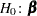=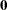. Let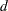be the number of clusters (or observations) minus the number of strata (or one) andbe the number of estimable parameters in the analysis model.

The likelihood ratio test is expressed as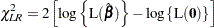where L() denotes the partial pseudo-likelihood described in Partial Likelihood Function for the Cox Model. The p-value is computed by using a chi-square distribution withdegrees of freedom. The usual assumptions required for a likelihood ratio test do not hold for the pseudo-likelihood that is used by PROC SURVEYPHREG, leading to other methods for testing the global null hypothesis, such as the Wald test discussed below.

Wald’s test is expressed as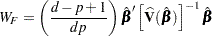The p-value is computed by using an F distribution with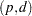degrees of freedom. For the Taylor series linearization method, the DF=PARMADJ option in the MODEL statement computes the p-value by using an F distribution with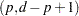degrees of freedom.

If you specify the DF=NONE option in the MODEL statement, then the procedure computes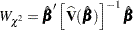and the p-value is computed by using a chi-square distribution withdegrees of freedom.